# Measurable decomposition

(diff) ← Older revision | Latest revision (diff) | Newer revision → (diff)
measurable partition, of a measure space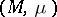A partition (cf. Decomposition)of the space into disjoint subsets (called the elements of the partition) that can be obtained as the partition into level sets of some measurable function (with numerical values) on. This definition can be restated in terms of "intrinsic" properties of the partition (see ). In accordance with the general tendency in questions of measure theory to ignore sets of measure zero, a measurable partition is often understood to be a partition measurable modulo 0, that is, equivalent modulo 0 to some measurable partition (two partitionsandof a measure spaceare equivalent modulo 0 if there exists a setof measure 0 such that the partitions of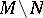consisting of the intersections withof the elements ofandcoincide).
Although the definition given makes sense for any measure space, in fact measurable partitions are almost always considered for Lebesgue spaces (cf. Lebesgue space) (and sometimes for spaces having the properties of the latter to some extent, for example, for spaces with perfect measures; see  and  and Perfect measure), since in these spaces measurable partitions have a number of "good" properties. Thus, in this case there exists a system of conditional measures (or, as used to be said in earlier times , a canonical system of measures) belonging to measurable partitions. This is a system of measures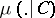on the elementsof the partitionwhich enables one to consider integration with respect toas repeated integration: first, integration is performed over theand with respect to appropriate, and then it is necessary to integrate the result, which can be looked upon as a function on the quotient space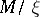, with respect to the natural measureon the latter space (by definition,has the elements ofas points, while its measurable subsets are those with measurable pre-images under the natural projection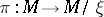; the measure is defined to be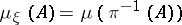). Interpretingas a space of elementary events in probability theory, one can say that the system of conditional measures is an "improvement" of the conditional probability, closely connected with the specific Lebesgue space; for arbitrary spaces of elementary events, the conditional probability cannot, in general, be interpreted using some set of measures on the elements of some partition.